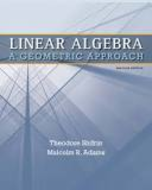### Solution Found!# Find a parametric equation of each of the following lines: a. 3x1 + 4x2 = 6 b. the line

Chapter 1, Problem 6

(choose chapter or problem)

Get Unlimited Answers! Check out our subscriptions
QUESTION:

Find a parametric equation of each of the following lines:

a. $$3 x_{1}+4 x_{2}=6$$

b. the line with slope 1/3 that passes through $$A=(-1,2)$$

c. the line with slope 2/5 that passes through $$A=(3,1)$$

d. the line through $$A=(-2,1)$$ parallel to $$\mathbf{x}=(1,4)+t(3,5)$$

e. the line through $$A=(-2,1)$$ perpendicular to $$\mathbf{x}=(1,4)+t(3,5)$$

f. the line through $$A=(1,2,1)$$ and $$B=(2,1,0)$$

g. the line through $$A=(1,-2,1)$$ and $$B=(2,1,-1)$$

h. the line through $$(1,1,0,-1)$$ parallel to $$\mathbf{x}=(2+t, 1-2 t, 3 t, 4-t)$$

### Questions & Answers (2 Reviews)

QUESTION:

Find a parametric equation of each of the following lines:

a. $$3 x_{1}+4 x_{2}=6$$

b. the line with slope 1/3 that passes through $$A=(-1,2)$$

c. the line with slope 2/5 that passes through $$A=(3,1)$$

d. the line through $$A=(-2,1)$$ parallel to $$\mathbf{x}=(1,4)+t(3,5)$$

e. the line through $$A=(-2,1)$$ perpendicular to $$\mathbf{x}=(1,4)+t(3,5)$$

f. the line through $$A=(1,2,1)$$ and $$B=(2,1,0)$$

g. the line through $$A=(1,-2,1)$$ and $$B=(2,1,-1)$$

h. the line through $$(1,1,0,-1)$$ parallel to $$\mathbf{x}=(2+t, 1-2 t, 3 t, 4-t)$$

Step 1 of  9

Parametric equations have the form,

$$x=x_{0}+t v$$

### Review this written solution for 963693) viewed: 440 isbn: 9781429215213 | Linear Algebra: A Geometric Approach - 2 Edition - Chapter 1.1 - Problem 6

Thank you for your recent purchase on StudySoup. We invite you to provide a review below, and help us create a better product.

Textbook: Linear Algebra: A Geometric Approach

Click to rate

Write a review below (optional):

### Review this written solution for 963693) viewed: 440 isbn: 9781429215213 | Linear Algebra: A Geometric Approach - 2 Edition - Chapter 1.1 - Problem 6

Thank you for your recent purchase on StudySoup. We invite you to provide a review below, and help us create a better product.

Textbook: Linear Algebra: A Geometric Approach

Click to rate

Write a review below (optional):